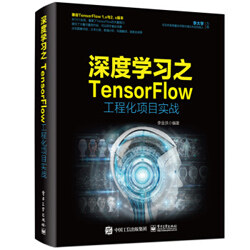# TensorFlow 工程实战（四）：使用带注意力机制的模型分析评论者是否满意## 一、熟悉样本：了解 tf.keras 接口中的电影评论数据集

IMDB 数据集中含有 25000 条电影评论，从情绪的角度分为正面、负面两类标签。该数据集相当于图片处理领域的 MNIST 数据集，在 NLP 任务中经常被使用。

def load_data(path='imdb.npz',  	#默认的数据集文件              num_words=None,			#单词数量，即文本转向量后的最大索引              skip_top=0,				#跳过前面频度最高的几个词              maxlen=None,			#只取小于该长度的样本              seed=113,				#乱序样本的随机种子              start_char=1,			#每一组序列数据最开始的向量值。              oov_char=2,				#在字典中，遇到不存在的字符用该索引来替换              index_from=3,			#大于该数的向量将被认为是正常的单词              **kwargs):				#为了兼容性而设计的预留参数

• (x_train, y_train)：训练数据集。如果指定了 num_words 参数，则最大索引值是 num_words-1。如果指定了 maxlen 参数，则序列长度大于 maxlen 的样本将被过滤掉。
• (x_test, y_test)：测试数据集。

## 二、代码实现：将 tf.keras 接口中的 IMDB 数据集还原成句子

• 加载 IMDB 数据集及字典：用 load_data 函数下载数据集，并用 get_word_index 函数下载字典。
• 读取数据并还原句子：将数据集加载到内存，并将向量转换成字符。

### 1. 加载 IMDB 数据集及字典

（1）找到 tf.keras.datasets.imdb 模块的源代码文件。以作者本地路径为例，具体如下：
C:\local\Anaconda3\lib\site-packages\tensorflow\python\keras\datasets\ imdb.py

#  origin_folder = 'https://storage.googleapis.com/tensorflow/tf-keras-datasets/'#  path = get_file(#      path,#      origin=origin_folder + 'imdb.npz',#      file_hash='599dadb1135973df5b59232a0e9a887c')

（3）在 get_word_index 函数中，将代码第 144~148 行注释掉。具体代码如下：

#  origin_folder = 'https://storage.googleapis.com/tensorflow/tf-keras-datasets/'#  path = get_file(#      path,#      origin=origin_folder + 'imdb_word_index.json',#      file_hash='bfafd718b763782e994055a2d397834f')

### 2. 读取数据并还原其中的句子

from __future__ import print_functionimport tensorflow as tfimport numpy as npattention_keras = __import__("8-10  keras 注意力机制模型 ") #定义参数num_words = 20000maxlen = 80batch_size = 32 #加载数据print('Loading data...')(x_train, y_train), (x_test, y_test) =  tf.keras.datasets.imdb.load_data(path='./imdb.npz',num_words=num_words)print(len(x_train), 'train sequences')print(len(x_test), 'test sequences')print(x_train[:2])print(y_train[:10])word_index = tf.keras.datasets.imdb.get_word_index('./imdb_word_index.json')#生成字典：单词与下标对应reverse_word_index = dict([(value, key) for (key, value) in word_index.items()])#生成反向字典：下标与单词对应 decoded_newswire = ' '.join([reverse_word_index.get(i - 3, '?') for i in x_train]) print(decoded_newswire)

（1）数据集大小为 25000 条样本。具体内容如下：

25000 train sequences25000 test sequences

（2）数据集中第 1 条样本的内容。具体内容如下：

[1, 14, 22, 16, 43, 530, 973, 1622, 1385, 65, 458, 4468, 66, 3941, 4, 173, 36, 256, 5, 25, 100, ……15, 297, 98, 32, 2071, 56, 26, 141, 6, 194, 7486, 18, 4, 226, 22, 21, 134, 476, 26, 480, 5, 144, 30, 5535, 18, 51, 36, 28, 224, 92, 25, 104, 4, 226, 65, 16, 38, 1334, 88, 12, 16, 283, 5, 16, 4472, 113, 103, 32, 15, 16, 5345, 19, 178, 32]

（3）前 10 条样本的分类信息。具体内容如下：

[1 0 0 1 0 0 1 0 1 0]

（4）第 1 条样本数据的还原语句。具体内容如下：

? this film was just brilliant casting location scenery story direction everyone’s really suited the part they played and you could just imagine being there robert ? is an amazing actor and now the …… someone’s life after all that was shared with us all

## 三、代码实现：用 tf.keras 接口开发带有位置向量的词嵌入层

（1）将自己的层定义成类，并继承 tf.keras.layers.Layer 类。
（2）在类中实现 __init__ 方法，用来对该层进行初始化。
（3）在类中实现 build 方法，用于定义该层所使用的权重。
（5）在类中实现 compute_output_shape 方法，指定该层最终输出的 shape。

import tensorflow as tffrom tensorflow import kerasfrom tensorflow.keras import backend as K 		#载入 keras 的后端实现 class Position_Embedding(keras.layers.Layer):  	#定义位置向量类      def __init__(self, size=None, mode='sum', **kwargs):        self.size = size #定义位置向量的大小，必须为偶数，一半是 cos，一半是 sin        self.mode = mode        super(Position_Embedding, self).__init__(**kwargs)            def call(self, x): 							#实现调用方法        if (self.size == None) or (self.mode == 'sum'):            self.size = int(x.shape[-1])        position_j = 1. / K.pow(  10000., 2 * K.arange(self.size / 2, dtype='float32') / self.size  )        position_j = K.expand_dims(position_j, 0)        #按照 x 的 1 维数值累计求和，生成序列。        position_i = tf.cumsum(K.ones_like(x[:,:,0]), 1)-1         position_i = K.expand_dims(position_i, 2)        position_ij = K.dot(position_i, position_j)        position_ij = K.concatenate([K.cos(position_ij), K.sin(position_ij)], 2)        if self.mode == 'sum':            return position_ij + x        elif self.mode == 'concat':            return K.concatenate([position_ij, x], 2)            def compute_output_shape(self, input_shape): #设置输出形状        if self.mode == 'sum':            return input_shape        elif self.mode == 'concat':        return (input_shape, input_shape, input_shape+self.size)

• 加和方式：通过 sum 运算，直接把位置向量加到原有的词嵌入中。这种方式不会改变原有的维度。
• 连接方式：通过 concat 函数将位置向量与词嵌入连接到一起。这种方式会在原有的词嵌入维度之上扩展出位置向量的维度。

## 四、代码实现：用 tf.keras 接口开发注意力层

class Attention(keras.layers.Layer):			#定义注意力机制的模型类    def __init__(self, nb_head, size_per_head, **kwargs):        self.nb_head = nb_head  				#设置注意力的计算次数 nb_head        #设置每次线性变化为 size_per_head 维度        self.size_per_head = size_per_head        self.output_dim = nb_head*size_per_head 	#计算输出的总维度        super(Attention, self).__init__(**kwargs)     def build(self, input_shape):				#实现 build 方法，定义权重        self.WQ = self.add_weight(name='WQ',                           shape=(int(input_shape[-1]), self.output_dim),                          initializer='glorot_uniform',                          trainable=True)        self.WK = self.add_weight(name='WK',                           shape=(int(input_shape[-1]), self.output_dim),                          initializer='glorot_uniform',                          trainable=True)        self.WV = self.add_weight(name='WV',                           shape=(int(input_shape[-1]), self.output_dim),                          initializer='glorot_uniform',                          trainable=True)        super(Attention, self).build(input_shape)    #定义 Mask 方法，按照 seq_len 的实际长度对 inputs 进行计算    def Mask(self, inputs, seq_len, mode='mul'):         if seq_len == None:            return inputs        else:            mask = K.one_hot(seq_len[:,0], K.shape(inputs))            mask = 1 - K.cumsum(mask, 1)            for _ in range(len(inputs.shape)-2):                mask = K.expand_dims(mask, 2)            if mode == 'mul':                return inputs * mask            if mode == 'add':                return inputs - (1 - mask) * 1e12                    def call(self, x):        if len(x) == 3:						#解析传入的 Q_seq、K_seq、V_seq            Q_seq,K_seq,V_seq = x            Q_len,V_len = None,None			#Q_len、V_len 是 mask 的长度        elif len(x) == 5:					            Q_seq,K_seq,V_seq,Q_len,V_len = x                    #对 Q、K、V 做线性变换，一共做 nb_head 次，每次都将维度转化成 size_per_head         Q_seq = K.dot(Q_seq, self.WQ)        Q_seq = K.reshape(Q_seq, (-1, K.shape(Q_seq), self.nb_head, self.size_per_head))        Q_seq = K.permute_dimensions(Q_seq, (0,2,1,3)) #排列各维度的顺序。        K_seq = K.dot(K_seq, self.WK)        K_seq = K.reshape(K_seq, (-1, K.shape(K_seq), self.nb_head, self.size_per_head))        K_seq = K.permute_dimensions(K_seq, (0,2,1,3))        V_seq = K.dot(V_seq, self.WV)        V_seq = K.reshape(V_seq, (-1, K.shape(V_seq), self.nb_head, self.size_per_head))        V_seq = K.permute_dimensions(V_seq, (0,2,1,3))        #计算内积，然后计算 mask，再计算 softmax        A = K.batch_dot(Q_seq, K_seq, axes=[3,3]) / self.size_per_head**0.5        A = K.permute_dimensions(A, (0,3,2,1))        A = self.Mask(A, V_len, 'add')        A = K.permute_dimensions(A, (0,3,2,1))            A = K.softmax(A)        #将 A 再与 V 进行内积计算        O_seq = K.batch_dot(A, V_seq, axes=[3,2])        O_seq = K.permute_dimensions(O_seq, (0,2,1,3))        O_seq = K.reshape(O_seq, (-1, K.shape(O_seq), self.output_dim))        O_seq = self.Mask(O_seq, Q_len, 'mul')        return O_seq            def compute_output_shape(self, input_shape):        return (input_shape, input_shape, self.output_dim) 

• input_shape 是 Q、K、V 中对应角色的输入维度。
• output_dim 是输出的总维度，即注意力的运算次数与每次输出的维度乘积（见代码 36 行）。

（1）对注意力机制中的三个角色的输入 Q、K、V 做线性变化（见代码第 45~53 行，书中第 75～83 行）。
（2）调用 batch_dot 函数，对第（1）步线性变化后的 Q 和 K 做基于矩阵的相乘计算（见代码第 55~59 行，书中第 85～89 行）。
（3）调用 batch_dot 函数，对第（2）步的结果与第（1）步线性变化后的 V 做基于矩阵的相乘计算（见代码第 55~59 行，书中第 85～89 行）。

## 五、代码实现：用 tf.keras 接口训练模型

（1）用 Model 类定义一个模型，并设置好输入 / 输出的节点。
（2）用 Model 类中的 compile 方法设置反向优化的参数。
（3）用 Model 类的 fit 方法进行训练。

#数据对齐x_train =  tf.keras.preprocessing.sequence.pad_sequences(x_train, maxlen=maxlen)x_test =  tf.keras.preprocessing.sequence.pad_sequences(x_test, maxlen=maxlen)print('Pad sequences x_train shape:', x_train.shape)	#定义输入节点S_inputs = tf.keras.layers.Input(shape=(None,), dtype='int32') #生成词向量embeddings = tf.keras.layers.Embedding(num_words, 128)(S_inputs)embeddings = attention_keras.Position_Embedding()(embeddings) #默认使用同等维度的位置向量 #用内部注意力机制模型处理O_seq = attention_keras.Attention(8,16)([embeddings,embeddings,embeddings]) #将结果进行全局池化O_seq = tf.keras.layers.GlobalAveragePooling1D()(O_seq)#添加 dropoutO_seq = tf.keras.layers.Dropout(0.5)(O_seq)#输出最终节点outputs = tf.keras.layers.Dense(1, activation='sigmoid')(O_seq)print(outputs)#将网络结构组合到一起model = tf.keras.models.Model(inputs=S_inputs, outputs=outputs) #添加反向传播节点model.compile(loss='binary_crossentropy',optimizer='adam', metrics=['accuracy']) #开始训练print('Train...')model.fit(x_train, y_train, batch_size=batch_size,epochs=5, validation_data=(x_test, y_test))

## 六、运行程序

Epoch 1/525000/25000 [==============================] - 42s 2ms/step - loss: 0.5357 - acc: 0.7160 - val_loss: 0.5096 - val_acc: 0.7533Epoch 2/525000/25000 [==============================] - 36s 1ms/step - loss: 0.3852 - acc: 0.8260 - val_loss: 0.3956 - val_acc: 0.8195Epoch 3/525000/25000 [==============================] - 36s 1ms/step - loss: 0.3087 - acc: 0.8710 - val_loss: 0.4135 - val_acc: 0.8184Epoch 4/525000/25000 [==============================] - 36s 1ms/step - loss: 0.2404 - acc: 0.9011 - val_loss: 0.4501 - val_acc: 0.8094Epoch 5/525000/25000 [==============================] - 35s 1ms/step - loss: 0.1838 - acc: 0.9289 - val_loss: 0.5303 - val_acc: 0.8007